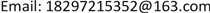1. 引言

2. 预备知识

⌊ x ⌋ ≤ x ； ⌈ x ⌉ ≥ x ； ⌊ x ⌋ = x ⇔ x是一个整数 ⇔ ⌈ x ⌉ = x 。

⌊ x ⌋ − ⌈ x ⌉ = 1 ; x − 1 < ⌊ x ⌋ ≤ ⌈ x ⌉ < x + 1 ; ⌊ − x ⌋ = − ⌈ x ⌉ ; ⌈ − x ⌉ = − ⌊ x ⌋

⌊ x ⌋ = n ⇔ n ≤ x < n + 1 ; ⌊ x ⌋ = n ⇔ x − 1 < n ≤ x ; ⌈ x ⌉ = n ⇔ n − 1 < x ≤ n ; ⌈ x ⌉ = n ⇔ x ≤ n < x + 1 ; ⌊ x + n ⌋ = ⌊ x ⌋ + n ; ⌈ x + n ⌉ = ⌈ x ⌉ + n

x < n ⇔ ⌊ x ⌋ < n ; n < x ⇔ n < ⌈ x ⌉ ; x ≤ n ⇔ ⌈ x ⌉ ≤ n ; n ≤ x ⇔ n ≤ ⌊ x ⌋

⌊ f ( x ) ⌋ = ⌊ f ( ⌊ x ⌋ ) ⌋

⌈ f ( x ) ⌉ = ⌈ f ( ⌈ x ⌉ ) ⌉

⌊ ⌊ x ⌋ ⌋ = ⌊ x ⌋ ; ⌈ x ⌉ = ⌈ x ⌉

3. 问题的提出

1) 易知，一次购买10瓶时，按“4个啤酒瓶换1瓶”的规定，可换3瓶啤酒。如果一次购买20瓶时，可换多少瓶啤酒？会是6瓶吗？

2) 当一次购买的数量很多时，比如，1000瓶、99,999瓶，如何算出可换多少瓶啤酒？

3) 假设每瓶啤酒的价格是a元，则花10a元可得12瓶，平均每瓶合10a/12元，小于a元/瓶。

4) 从理论上讲，当一次购买的数量为无穷大时，可换啤酒量占购买量的比会是一个常数吗？

5) 从理论上讲，当一次购买的数量为无穷大时，按照规定，商家会赔吗？平均每瓶啤酒的价格会降到0吗？如不是，会是多少？

6) 假设现有900人，每人只喝3瓶啤酒，按照规定，请问一次购买多少瓶即可满足每人3瓶？

4. 问题的探究

1) 数学模型的建立

⌊ 1 + n − 4 3 ⌋ = ⌊ n − 1 3 ⌋ 。

2) 当购买很多啤酒时，换得的啤酒数量ΔN占购买量n多少？

1 n ( n − 1 3 − 1 ) < 1 n ⌊ n − 1 3 ⌋ ≤ 1 n n − 1 3

1 n ( n − 4 3 ) < 1 n ⌊ n − 1 3 ⌋ ≤ 1 n n − 1 3

lim n → ∞ 1 n n − 4 3 = lim n → ∞ 1 n n − 1 3 = 1 3

lim n → ∞ Δ N n = 1 3

Δ N n = 0.333333296

Δ N = ⌊ 10000000000 − 1 3 ⌋ = ⌊ 3333333333 ⌋ = 3333333333

Δ N n = 0.333333333

3) 由简单到复杂

4) 设k个空瓶换m瓶( k > m ，， k ∈ N ， m ∈ N 常数)。

1 n m n − m m − k + m k − m = 1 n ( m n − m m k − m − 1 ) < 1 n ⋅ ⌊ m n − m m k − m ⌋ ≤ 1 n ⋅ m n − m m k − m

lim n → ∞ n a n + Δ N = lim n → ∞ n a n + ⌊ m n − m m k − m ⌋ = lim n → ∞ n a n k − m m k − m = k − m k a

lim n → ∞ n ( a − b ) n + Δ N = lim n → ∞ n ( a − b ) n + ⌊ m n − m m k − m ⌋ = lim n → ∞ n ( a − b ) n k − m m k − m = k − m k ( a − b )

5. 几类应用

2700 ≤ ⌊ 4 n − 1 3 ⌋ n ≥ [ 2025.25 ] = 2026

y = n ⋅ ( 4 − 2 ) = 2026 × 2 = 4052

z = n ⋅ ( 4 − 1 4 ) ⋅ 4 = 6078

3 M ≤ [ n + Δ N ] = n + ⌊ n − 1 3 ⌋ = ⌊ 4 n − 1 3 ⌋

3 M ≤ 4 n − 1 3

y = n ⋅ ( 4 − 2 ) ≥ ( 9 M + 1 2 ) ( 4 − 2 ) = 9 M + 1 2

z = n ⋅ ( 4 − 1 4 ) ⋅ 4 ≥ 9 M + 1 4 × 3 = 27 M + 3 4

3 A ≤ n k − m m k − m 从而

n ≥ 3 A ( k − 1 ) k

y = n ⋅ ( 4 − 2 ) ≥ 3 A ( k − 1 ) k ⋅ 2 = 6 A ( k − 1 ) k

z = n ⋅ ( k − 1 k ) ⋅ 4 ≥ 3 A ( k − 1 ) k ⋅ k − 1 k = 12 A ( k − 1 ) 2 k 2

B ≤ n k − m 2 k − m ，从而

n ≥ B ( k − m ) + m 2 k

y = n ⋅ ( 4 − 2 ) = 2 B ( k − m ) + 2 m 2 k

z = 4 n ⋅ k − m k ≥ 4 B ( k − m ) 2 + 4 ( k − m ) k 2 k 2